Home > GHCN, trb, Uncategorized > trb-0.03: Weighted Latitudinal Zoning

## trb-0.03: Weighted Latitudinal Zoning

2010 July 12

Introduction

Changes in latitude, changes in attitude.

Weighted Latitudunal Zoning

The area between two latitudes, as a fraction of the sphere, is given by the simple formula
(sin(β) – sin(α))

In the previous post, we used 6 bands of 30 degrees.
Calculating the area in each band:

``` 90 to  60: 0.0669873
60 to  30: 0.1830127
30 to   0 : 0.2500000
0 to -30: 0.2500000
-30 to -60: 0.1830127
-60 to -90: 0.0669873```

You can see the numbers add up to “1”, which means that all the zones equal 100% of the area.

Let’s say the temp for each zone is as follows (just made up numbers):

``` 90 to  60: -4
60 to  30: 6
30 to   0 : 12
0 to -30: 10
-30 to -60: 8
-60 to -90: -10```

The simple mean of these values is
(-4 + 6 + 12 + 10 + 8 – 10) / 6 = 3.67

To calculate the weighted mean, we multiply each zone value by the zone fractional area and add them up:

```  ( -4 * 0.0669873)
+ (  6 * 0.1830127)
+ ( 12 * 0.2500000)
+ ( 10 * 0.2500000)
+ (  8 * 0.1830127)
+ (-10 * 0.0669873)
= 7.124356```

Code

```###############################################################################
# trb 0.03 : 2010 07 12 : Weighted Latitudinal Bands
# trb 0.02 : 2010 07 11 : Simple Latitudinal Bands
# trb 0.01 : 2010 07 10 : GHCN Simple Mean Average
# https://rhinohide.wordpress.com
###############################################################################

###############################################################################
###############################################################################
# ftp://ftp.ncdc.noaa.gov/pub/data/ghcn/v2/v2.mean
# ftp://ftp.ncdc.noaa.gov/pub/data/ghcn/v2/v2.temperature.inv
###############################################################################

#------------------------------------------------------------------------------
# Temperature File (GHCN formatted monthly means)
#------------------------------------------------------------------------------

# mm for 'monthly means'
mmwids=c(11,1,4,5,5,5,5,5,5,5,5,5,5,5,5)
mmcols=c("id","dupe","year","jan","feb","mar","apr","may","jun",
"jul","aug","sep","oct","nov","dec")

#------------------------------------------------------------------------------
# Inventory File
#------------------------------------------------------------------------------

# there are five occurrances of the pound hash in v2.temperature.inv
# R scripts chokes on reading those, remove them first

invcols <- c("id","name","lat","lon","alt","elev","ru","pop","terr","arid",
invwids <- c(11,32,6,8,5,5,1,5,2,2,2,2,1,2,16,1,5)

###############################################################################
# Set up arrays for latitudinal banding
###############################################################################

hindex=new.env(hash=TRUE)
hlat=new.env(hash=TRUE)
hlon=new.env(hash=TRUE)
for (i in 1:nrow(inv)) {
hindex[[as.character(inv[i,1])]] <- i
hlat[[as.character(inv[i,1])]] <- inv[i,3]
hlon[[as.character(inv[i,1])]] <- inv[i,4]
}

mmlat <- rep(NA,nrow(mm))
mmlon <- rep(NA,nrow(mm))

for (i in 1:nrow(mm)) {
mmlat[i] <- hlat[[as.character(mm[i,1])]]
mmlon[i] <- hlon[[as.character(mm[i,1])]]
}

# general formula for number of latitude bands
nbands <- 36
bands <- seq(90,-90,by=(-180/nbands))

# display the area of each latitude band
# l <- rep(NA,nbands)
# for (i in 1:nbands) {
#    l[i] <- abs((sin(bands[i]*pi/180) - sin(bands[i+1]*pi/180)))/2
# }
# sum(l) # should always be 1
# l

###############################################################################
# Calculate Annual Mean Average
###############################################################################

#------------------------------------------------------------------------------
# set up the range of years to calculate
#------------------------------------------------------------------------------
#year0 <- min(mm[,3])
year0 <- 1880
year1 <- max(mm[,3])
range <- year1 - year0 + 1

#------------------------------------------------------------------------------
# initialize some vectors to hold annual results
#------------------------------------------------------------------------------
lyear <- rep(NA, range)
lmean <- matrix(NA,range,nbands)
lcount <- matrix(NA,range,nbands)

#------------------------------------------------------------------------------
# loop through the range of years
#------------------------------------------------------------------------------
for (i in 1:range) {

year <- i + year0 -1

#------------------------------------------------------------------------------
# loop through the latitude bands
#------------------------------------------------------------------------------

for (j in 1:nbands) {

# select the latitude out of the mm list first
# since lat data is not embedded in mm and we depend on symmetry with mmlt
lmax <- bands[j]
lmin <- bands[j+1]
idx=(mmlat<=lmax & mmlat>=lmin)
mmlt=mm[idx,]

# select the year out of the mmlt
idx=(mmlt[,3]==year)
mmyr=mmlt[idx,]

# calculate the annual means
# divide by 10 since means are stored as 10ths of T

# fractional area for this latitudinal band
fa <- abs((sin(lmax*pi/180) - sin(lmin*pi/180)))/2

# only use years with data for every month
#lmean[i,j]= (mean(rowMeans(mmyr[,4:15]),na.rm=T)/10) * fa

# use every year, even those with partial data
lmean[i,j]= ( mean(rowMeans(mmyr[,4:15],na.rm=T),na.rm=T)/10 ) * fa
lcount[i,j]=nrow(mmyr)
}
year[i]=year
}
#------------------------------------------------------------------------------
# calculate the global land temperature
#------------------------------------------------------------------------------
# previously we took the mean which weights each lat evenly
# ameans = rowMeans(lmean,na.rm=T) # mean(ameans, na.rm=T)
# 10.48877
# but now each zone has already been multiplied by a fractional weight
# so we just add the zones together
# but what if some zones are NA? For now, nothing
ameans = rowSums(lmean,na.rm=T)
mean(ameans, na.rm=T)
# nband= 4, 12.42837
# nband= 6, 15.28229
# nband=32, 14.81797
# nband=36, 14.74784
# nband=36, 14.77640 (partial years)
###############################################################################
# Display
###############################################################################
# plot some stuff
# do this nband times
for (i in 1:nbands) {
if ( length(lmean[!is.na(lmean[,i]),i]) > 0 ) {
img=paste("trb-003-zone-",i,".png", sep="")
tit=paste("Latitudes",bands[i],"to",bands[i+1], sep=" ")
png(img, width=600, height=150)
par(mar=c(2, 2, 2, 1))
plot(lmean[,i] ~ lyear, ylab="Temp (C)", xlab="", type="l",
main=tit, cex.main=0.8)
}
}

# to avoid the 0 for 2010, drop last value
img=paste("trb-003-zone-all.png", sep="")
png(img, width=600, height=150)
par(mar=c(2, 2, 2, 1))
plot (ameans[1:(length(ameans)-1)] ~ lyear[1:(length(ameans)-1)], type="l", xlab="", ylab="Temp (C)",
main="GHCN Weighted Latitudinal Averaged Temps",
sub="https://rhinohide.wordpress.com (trb-0.03)")```

Results

First, a list of the station counts by year for each band
http://rhinohide.org/rhinohide.cx/co2/trb/0.03/b36-partial/trb-003-station-count-36-bands.csv

Here are the charts for 36 bands which is a 5deg step between latitudes. Two charts are not available since there was no station data for the entire span of years.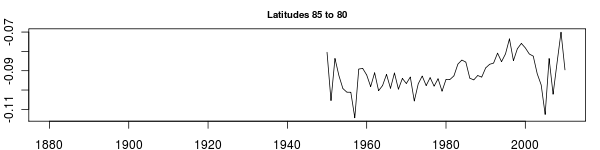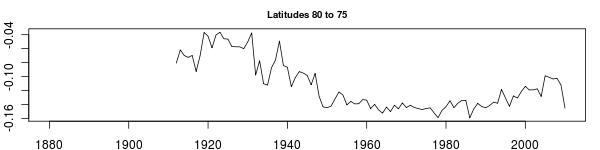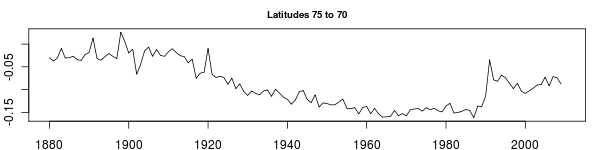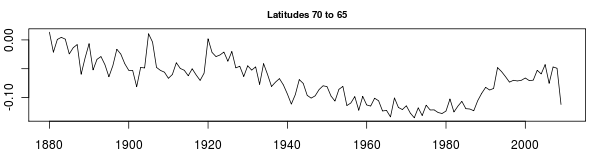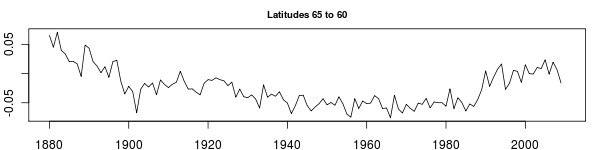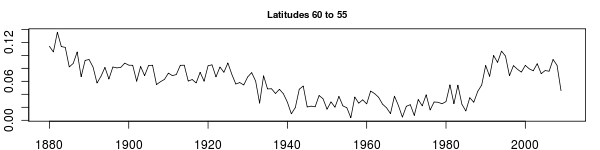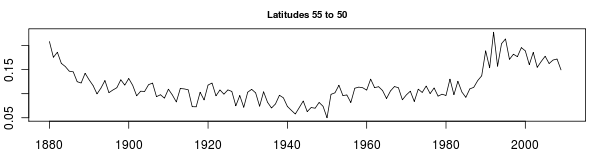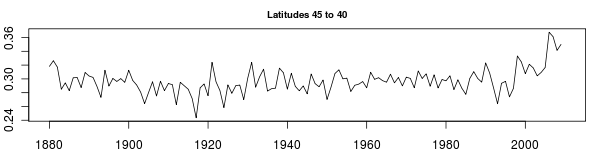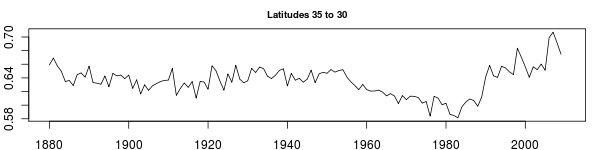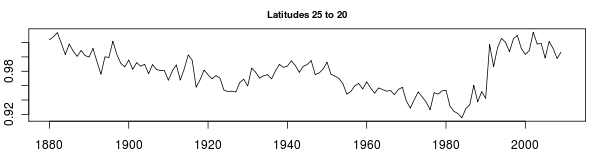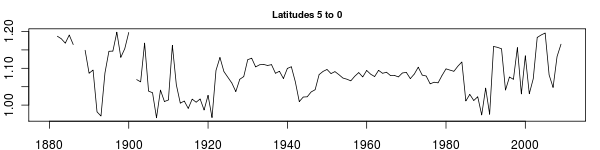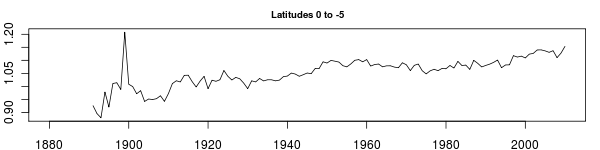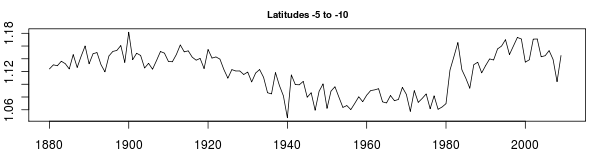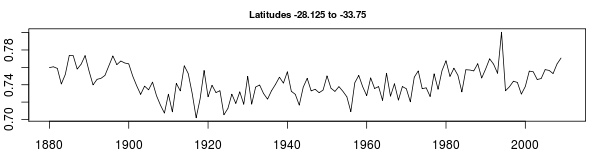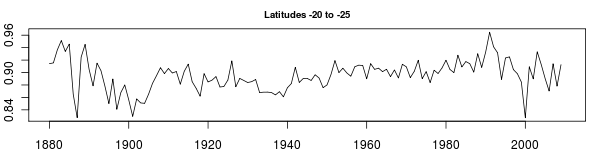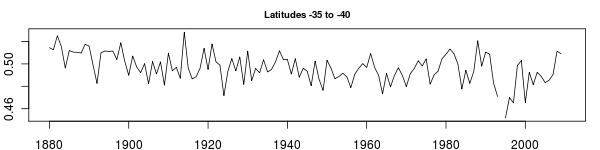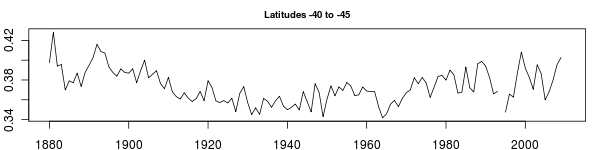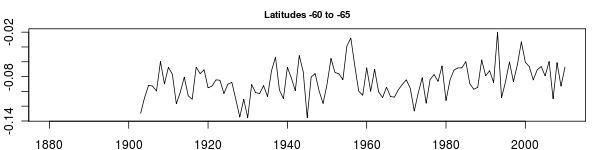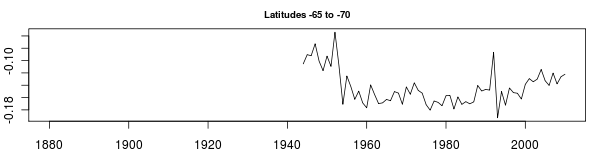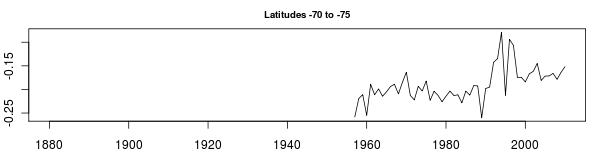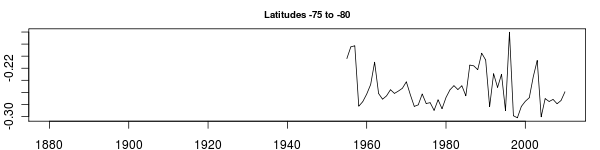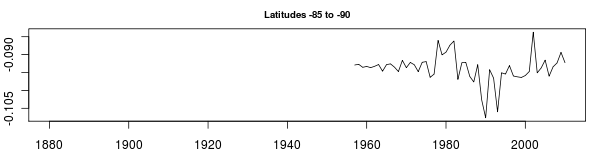But now we have some empty zones. Since we are summing over the zones, how do we adjust for the missing ones. A simple reweighting doesn’t work, since the ave temps in each zone are different. We could interpolate over the zones, and sum the interpolation into the final temperature. We could use anomalies or trends to remove the latitude bias in temps and avoid the problem. Or we could go get more data from the oceans. Eventually, I’ll probably do all three.

1. 2010 July 12 at 8:43 am

that sudden dip around 2006 (?) on a lot of the panels looks odd.

There’s something to be said for using equal-area bands, instead of strict 5 degree bands.

2. 2010 July 12 at 9:33 am

Agreed.
Thought of that on the way to work this morning.
I’ll make the change in trb-0.04

3. 2010 July 12 at 9:49 am

you could just combine the polar bands. I know you’re concerned that the (empty) 85-90 deg band will behave differently from the 80-85 band and the 75-80 band, but with the data you’ve got, you just don’t have that information in the first place. You’ll have to smear it out, I think.

So I think it’d be reasonable to make one single band from 75-90 C, or something like that.

Or, you could leave the empty bands empty, as CRU does. This is the same as assuming that the empty bands do the same thing as the mean of the rest of the earth.

4. 2010 July 12 at 10:56 am

I did that on an earlier scratch version of the station set mean,
where I just aggregated some of the high lat zones.

bands=c(90,70,60,55,50,45 … -45,-50,-55,-60,-70,-90)

You can leave bands empty with anomalies … not so good with abs temps.
But as a general method, I prefer an algorithmic approach – either a common step size or a common area. That way you can increase or decrease the bands with ease.

I can understand why some might want a “mixed” solution, like these bands shown here. People generally like thinking in simple step sizes – so a 5x5deg grid seems obvious. They are less likely to *intuitively* grasp just how different the area of these grids are.

Now I want to violate KISS. Now I want to code flags for fixed step size, fixed area, or predefined mixed. But I will resist – for now.